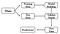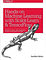# Build A Simple Stock Movement Classifier Using Machine Learning & Python

Can stock indicators combined with machine learning predict the price movement of stocks ?

Disclaimer: The material in this article is purely educational and should not be taken as professional investment advice. Invest at your own discretion.

Before we begin, if you enjoy my articles and content and would like more content on programming, stocks, machine learning, etc. , then please give this article a few claps, it definitely helps out and I truly appreciate it ! So let’s begin !

In this article I will attempt to create a model that can determine if the price of an asset will go up or down the next day based on stock data using machine learning, technical indicators and python ! It is extremely hard to try and predict the stock market momentum direction, but let’s give it a try.

# What Are Stock Market Technical Indicators ?

Stock market technical indicators are signals used to interpret stock or financial data trends to attempt to predict future price movements within the market. Stock indicators help investors to make trading decisions.

# Types of Technical Indicators

Simple Moving Average (SMA): A simple moving average is a technical trend indicator that can aid in determining if an asset price will continue or if it will reverse a bull or bear trend. A simple moving average can be enhanced as an exponential moving average (EMA) that is more heavily weighted on recent price action. -investopedia

Exponential Moving Average (EMA): The EMA is a moving average that places a greater weight and significance on the most recent data points. Like all moving averages, this technical trend indicator is used to produce buy and sell signals based on crossovers and divergences from the historical average. -investopedia

Moving Average Convergence Divergence (MACD) : Moving Average Convergence Divergence (MACD) is a trend-following momentum indicator that shows the relationship between two moving averages of a security’s price. The MACD is calculated by subtracting the 26-period Exponential Moving Average (EMA) from the 12-period EMA. -investopedia

Relative Strength Index (RSI): The relative strength index (RSI) is a momentum indicator used in technical analysis that measures the magnitude of recent price changes to evaluate overbought or oversold conditions in the price of a stock or other asset. -investopedia

These are the indicators that we will be programming in this article using python.

# What is Machine Learning ?

Machine learning is a subset of artificial intelligence, it is the science of getting computers to act without being explicitly programmed, and is mostly just statistics. Machine learning is used to find patterns in data that you can then make predictions on. It can be subdivided into supervised learning and unsupervised learning or some mixture of both.

Machine learning is a computer program said to learn from experience ‘E’ with respect to some class of tasks ‘T’ and performance measure ‘P’, if its performance at tasks in ‘T’, as measured by ‘P’, improves with experience ‘E’.
— Tom MitchellHow Machine Learning Works

Before writing any code, if you prefer not to read this article and would like a video representation of it, you can check out the YouTube Video . It goes through everything in this article with a little more detail, and will help make it easy for you to start programming even if you don’t have the programming language Python installed on your computer. Or you can use both as supplementary materials for learning !

# Programming

First, I want to create a description about the program so that I can simply read the description and know what the program is supposed to do or what the program is about.

`#Description: Use stock indicators with machine learning to try to predict the direction of a stock price: #1 means the stock price goes up #0 means the stock price goes down or stays the same`

Import the libraries that we will need throughout the program.

`#Import the librariesimport numpy as npimport pandas as pdfrom sklearn.model_selection import train_test_splitfrom sklearn.tree import DecisionTreeClassifier`

Load the data and store it into a variable. Note that I am using Google Collab to write this program, so I must use Googles library to upload the data set.

`#Load the data setfrom google.colab import filesfiles.upload()#Store the data into the data framedf = pd.read_csv('GOOG_Stock.csv')#show the data framedf`

# Create and Calculate the Indicators

Create functions to calculate the Simple Moving Average (SMA) and the Exponential Moving Average (EMA).

`#Create functions to calculate the SMA, & the EMA#Create the Simple Moving Average Indicator#Typical time periods for moving averages are 15, 20,& 30#Create the Simple Moving Average Indicatordef SMA(data, period=30, column='Close'):  return data[column].rolling(window=period).mean()#Create the Exponential Moving Average Indicatordef EMA(data, period=20, column='Close'):  return data[column].ewm(span=period, adjust=False).mean()`

Next, create a function to calculate the Moving Average Convergence Divergence (MACD).

`#Create a function to calculate the Moving Average Convergence/Divergence (MACD)def MACD(data, period_long=26, period_short=12, period_signal=9, column='Close'):    #Calculate the Short Term Exponential Moving Average    ShortEMA = EMA(data, period_short, column=column) #AKA Fast moving average    #Calculate the Long Term Exponential Moving Average    LongEMA = EMA(data, period_long, column=column) #AKA Slow moving average    #Calculate the Moving Average Convergence/Divergence (MACD)    data['MACD'] = ShortEMA - LongEMA    #Calcualte the signal line    data['Signal_Line'] = EMA(data, period_signal, column='MACD')#data['MACD'].ewm(span=period_signal, adjust=False).mean()            return data`

Last, but not least create a function to calculate the Relative Strength Index (RSI).

`#Create a function to calculate the Relative Strength Index (RSI)def RSI(data, period = 14, column = 'Close'):  delta = data[column].diff(1) #Use diff() function to find the discrete difference over the column axis with period value equal to 1  delta = delta.dropna() # or delta[1:]  up =  delta.copy() #Make a copy of this object’s indices and data  down = delta.copy() #Make a copy of this object’s indices and data  up[up < 0] = 0   down[down > 0] = 0   data['up'] = up  data['down'] = down  AVG_Gain = SMA(data, period, column='up')#up.rolling(window=period).mean()  AVG_Loss = abs(SMA(data, period, column='down'))#abs(down.rolling(window=period).mean())  RS = AVG_Gain / AVG_Loss  RSI = 100.0 - (100.0/ (1.0 + RS))    data['RSI'] = RSI  return data`

# Prepare the Data Set for Machine Learning

Add the indicators to the data set and show the data.

`#Add the indicators to the data set#Creating the data set MACD(df)RSI(df)df['SMA'] = SMA(df)df['EMA'] = EMA(df)#Show the datadf`

Create the target column.

`#Create the target columndf['Target'] = np.where(df['Close'].shift(-1) > df['Close'], 1, 0) # if tomorrows price is greater than todays price put 1 else put 0#Remove the date column#remove_list = ['Date'] #df = df.drop(columns=remove_list)#Show the datadf`

Remove the first 29 rows of data or days.

`#Remove the first 29 days of datadf = df[29:]#Show the data setdf`

Split the data set into a feature/independent data set (X) and a target/dependent data set (Y).

`#Split the data set into a feature or independent data set (X) and a target or dependent data set (Y)keep_columns = ['Close', 'MACD', 'Signal_Line', 'RSI', 'SMA', 'EMA']X = df[keep_columns].valuesY = df['Target'].values`

Split the data again, but this time into 80% training and 20% testing data sets.

`#Split the data again but this time into 80% training and 20% testing data setsX_train, X_test, Y_train, Y_test = train_test_split(X, Y, test_size = 0.2)`

# Create and Train the Machine Learning Model

Create and train the model.

`#Create and train the model tree = DecisionTreeClassifier().fit(X_train, Y_train)`

Check how well the model did on the training data.

`#Check how well the SVC Model on training dataprint(tree.score(X_train, Y_train))`

Check how well the model did on the testing data.

`#Check the SVC Model on the test data setprint(tree.score(X_test, Y_test))`

Get the classification report to see how well the model performed.

`from sklearn.metrics import classification_reportprint(classification_report(Y_test, rbf_svc_prediction))`

It looks like this model gave an accuracy score of about 68.18%. This model did better than guessing or flipping a coin which is encouraging, but with an accuracy level at 68.18% on this small set of data, it most certainly is not ready for real world trading, but this model is promising for exploring more on Machine Learning Classifiers for stock price movements. Maybe the model can be improved upon with the use of other indicators, more data, parameter tuning and more analysis.

If you want to start an investment portfolio, then sign up with WeBull using this link and get FREE stocks just for opening an account and funding it with an initial deposit of \$100 or more! It’s free stocks that you can either sell, play with or create your own trading strategy with. For a free stock trading app, I highly recommend it.

If you are interested in reading more on machine learning to immediately get started with problems and examples then I strongly recommend you check out Hands-On Machine Learning with Scikit-Learn and TensorFlow: Concepts, Tools, and Techniques to Build Intelligent Systems. It is a great book for helping beginners learn how to write machine learning programs, and understanding machine learning concepts.Hands-On Machine Learning with Scikit-Learn and TensorFlow: Concepts, Tools, and Techniques to Build Intelligent Systems

## More from randerson112358

A programmer that loves Computer Science: https://www.youtube.com/user/randerson112358 https://www.youtube.com/channel/UCbmb5IoBtHZTpYZCDBOC1